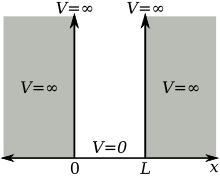# Infinite Well Problem - Time Independent Schrodinger's Equation

I'm currently taking a Semiconductor class and we're talking about Schrodinger's Wave Equation, specifically the 1 dimensional time independent form.

We were looking at the infinite potential well model:And we divided the graph into 3 different regions: first being the left (or negative) V(x)= -inf, the second being V(x)=0, and the third being V(x)= inf.

We solved the second region first using the equation:

d2$\Psi$(x)/dx2 + $\frac{2m}{\hbar}$*(E-V(x))*$\Psi$(x) = 0

Well my professor said this math should be something we could do easily, so pardon me if I seem a bit ignorant, but I really can't recall an effective way to tackle this problem. He gave us the solution, which is:

$\Psi$(x) = A1cos(kx) + A2sin(kx)

I should have included all the information needed, but if not please ask!

Thanks!